# 分数加法

### 加分数只需简单的三步：

• 一、确定下面的数（分母）相同
• 二、上面的数（分子）相加，把结果写在相同分母的上面（分子的位置）
• 三、约简分数（如需要）

## 例子 1:

1 4 + 1 4

、下面的数（分母）已经相同。跳到第二步。

、上面的数相加，把结果写在相同的分母上面：

1 4 + 1 4 = 1 + 1 4 = 2 4

、约简分数：

2 4 = 1 2

 1 4 + 1 4 = 2 4 = 1 2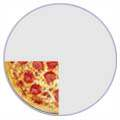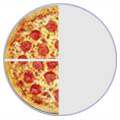。。。。。。看到 2 4 写成 1 2 会比较简单吗？（看等值分数。）

## 例子 2:

1 3 + 1 6

：下面的数不同。每块大小都不一样。

 1 3 + 1 6 = ？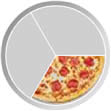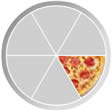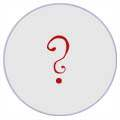"6" 是 "3"的两倍倍，所以要把下面的数变成一样的我们可以把第一个分数的上面和下面乘以2，像这样：

 × 2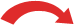1 3 = 2 6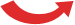× 2

 2 6 + 1 6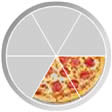：上面的数相加，写在相同的分母上面：

2 6 + 1 6 = 2 + 1 6 = 3 6

 2 6 + 1 6 = 3 6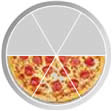：约简分数：

3 6 = 1 2

 2 6 + 1 6 = 3 6 = 1 2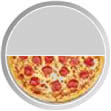### 用纸笔做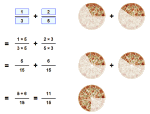"分数要加减

## 例子 3:

1 3 + 1 5

 1 3 + 1 5 = ？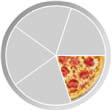5 15 + 3 15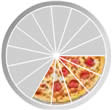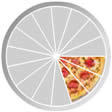× 51 3 = 5 15× 5

 × 31 5 = 3 15× 3

 5 15 + 3 15 = 8 15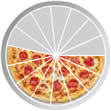## 把分母变成一样### 例子：杯形蛋糕

• 一个朋友可以给你材料，但你要给他销售额的1/3
• 租摊点要销售额的1/4

 1 + 1 = ？ 3 4 ?

1/3上面和下面的数乘以 4

 1 × 4 + 1 = ？ 3 × 4 4 ？

1/4上面和下面的数都乘以 3

 1 × 4 + 1 × 3 = ? 3 × 4 4 × 3 ？

 4 + 3 = 4+3 = 7 12 12 12 12